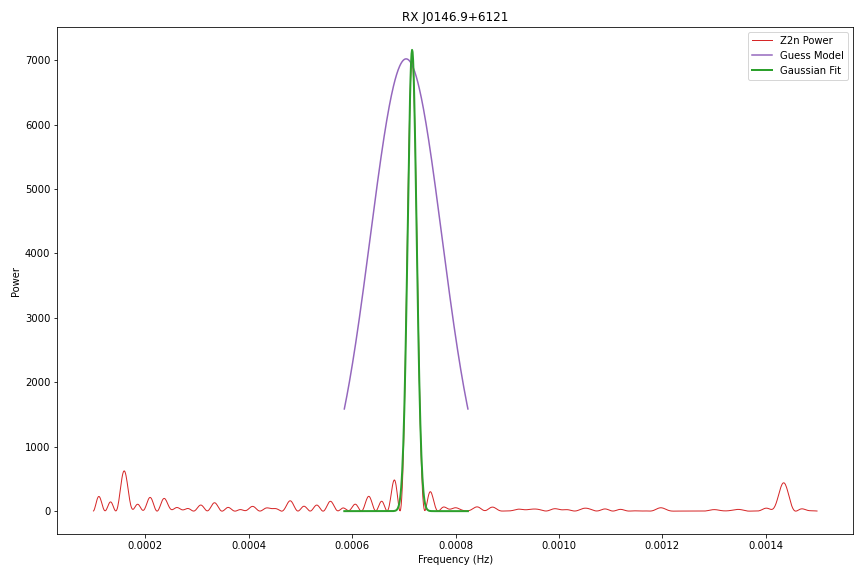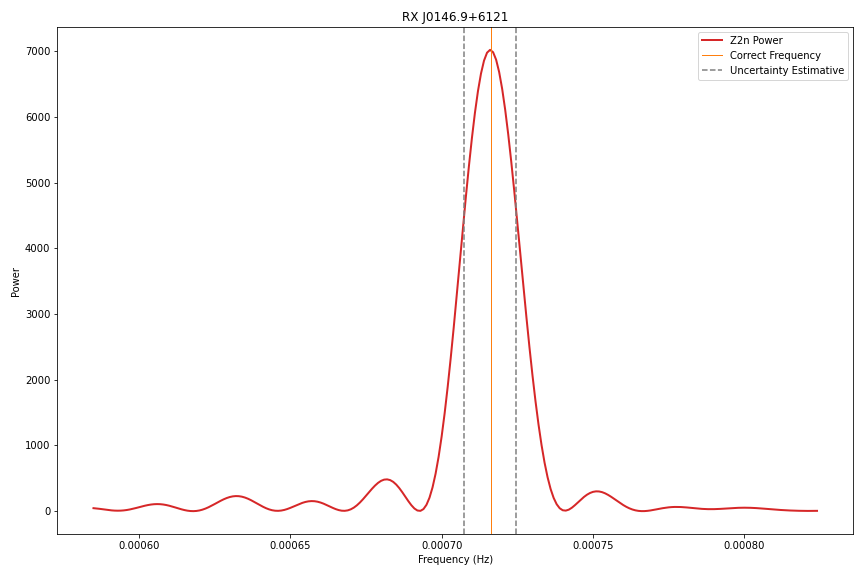# Determining uncertainty

The $Z^2_n$ Software provides an estimative of uncertainty on the selected peak region, based on the $\sigma$ of a gaussian like $g(x)$, function that is fitted with the least-squares method. The best fit in the least-squares sense minimizes the sum of squared residuals (a residual being: the difference between an observed value, and the fitted value provided by a model).

$\LARGE g(x) = {\mathrm{e}^{-\frac{(x - \overline{x}) ^ 2}{2 \cdot \sigma ^ 2}}}$

The complete process can be visualized on the next figure:The bandwith limited by the estimated uncertainty would look like this:# Pulsed Fraction

The $Z^2_n$ Software also provides the estimative of the pulsed fraction on the selected peak region, given by the equation wich is defined as follows.

$\Large f_p (\%) = (2 \cdot \frac{Z^2_n}{N}) ^ \frac{1}{2}$